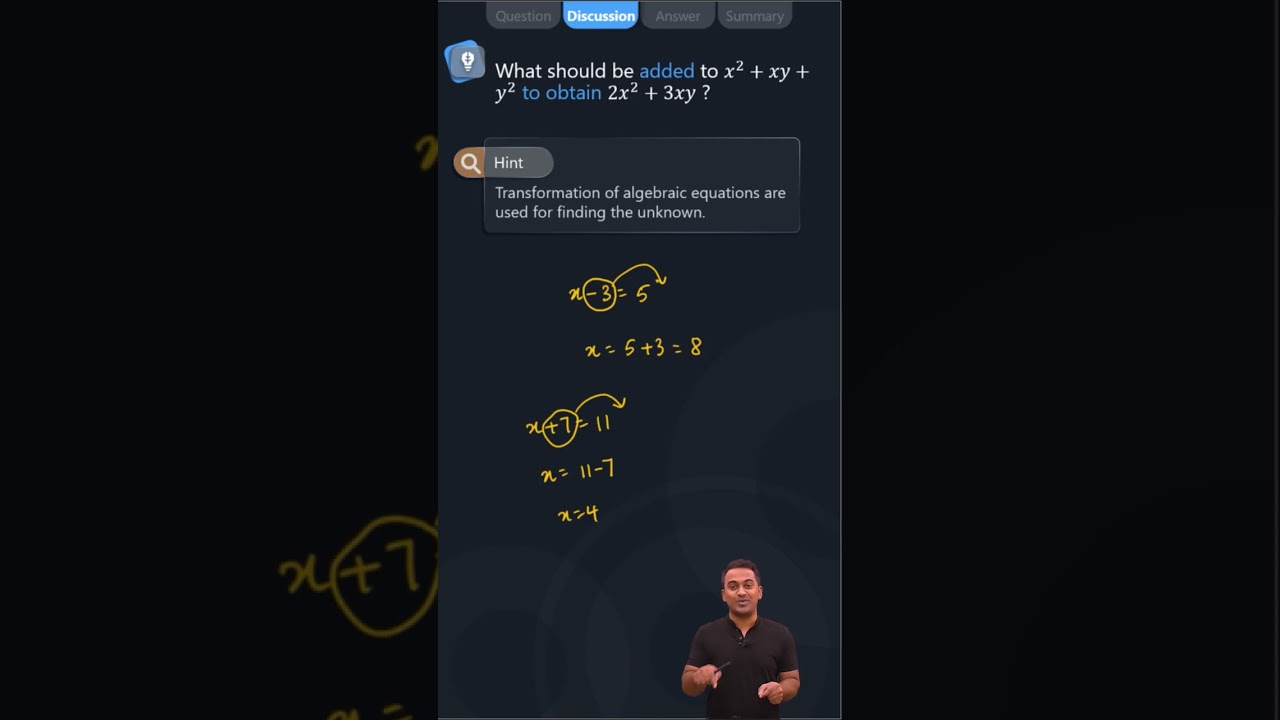What should be added to x2 + xy + y2 to obtain 2x2 + 3xy?Let us assume p be the required term

Then,

p + (x2 + xy + y2) = 2x2 + 3xy

p = (2x2 + 3xy) – (x2 + xy + y2)

p = 2x2 + 3xy – x2 – xy – y2

p = 2x2 – x2 + 3xy – xy – y2

p = x2 + 2xy – y2(2)(0)